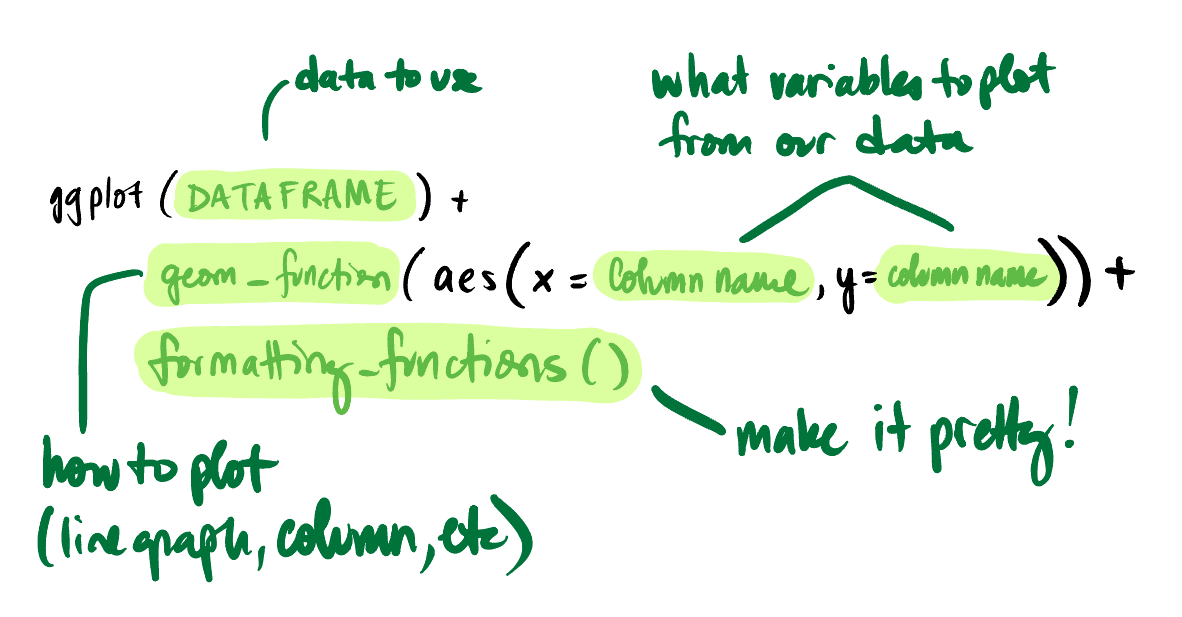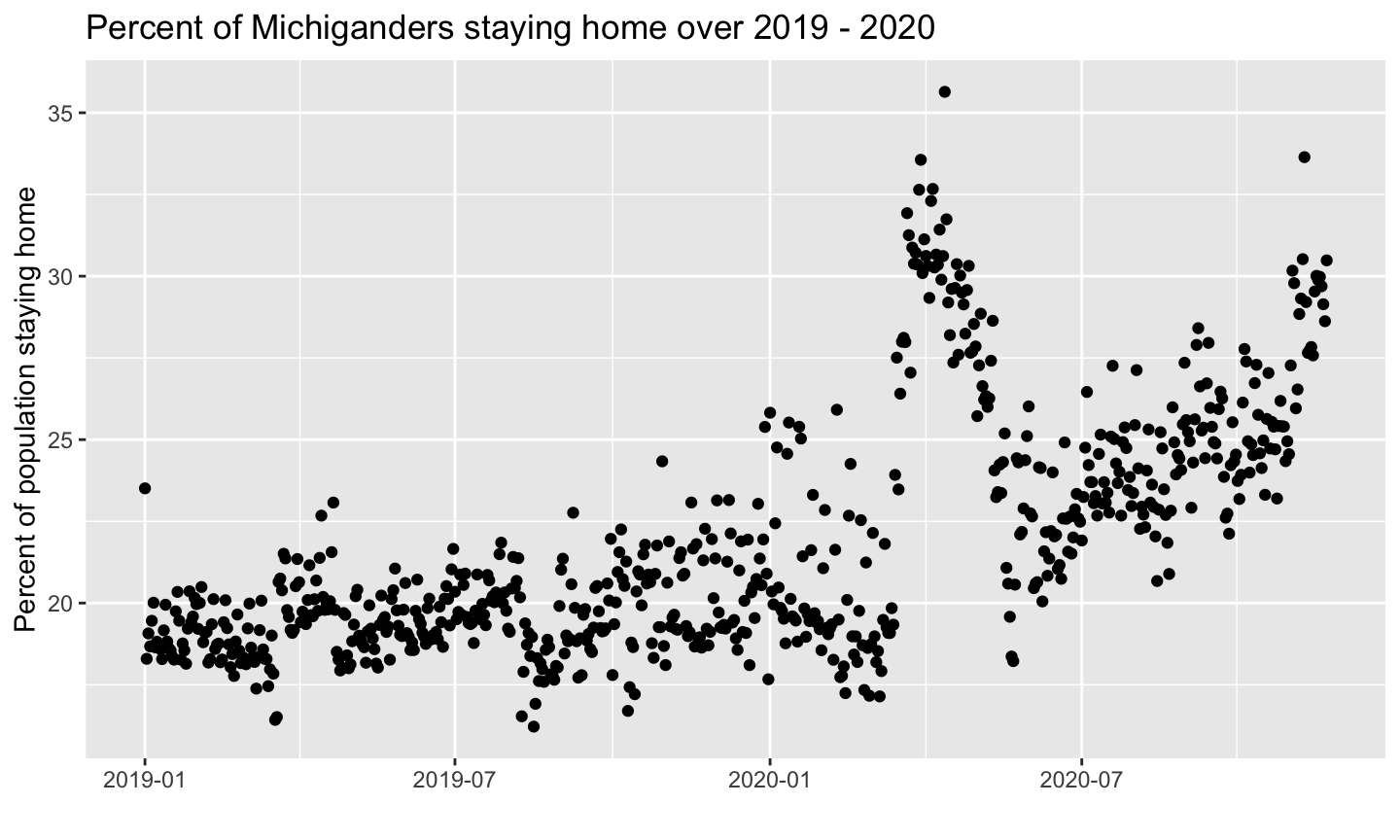2023-06-01

## `ggplot` basics

• `ggplot` follows a “grammar of graphics” that is a little different from the rest of R’s coding structure
• Basic components of a ggplot: a dataset (dataframe), aesthetics (`aes`), geoms, and formatting
• `ggplot` takes dataframes as the basic input, not an x vector and y vector
• `geom`’s are different types of plot objects that you can add to the plot (e.g. points, lines, bars, etc.)
• The `aes` (short for aesthetics) command tells ggplot which variables in the dataset represent the x values, y values, color, size, etc.
• You can set aes either in the main `ggplot` call or within a `geom`
• The official ggplot cheatsheet is great! Use this as a quick reference once you get the general idea

## `ggplot` basicsLet’s try an example!

## Simple example - Bureau of Transportation Statistics mobility data

Try running this code for yourself! (Be sure you have downloaded the datasets folder and placed it in your working directory first!)

```library(ggplot2)

# Calculate the percent of the population staying home
mobilityData\$PercentHome =
100 * mobilityData\$`Population Staying at Home` /
(mobilityData\$`Population Staying at Home` + mobilityData\$`Population Not Staying at Home`)```

## Plot code

```ggplot(mobilityData, aes(x = Date, y = PercentHome)) +

geom_point() +

labs(title="Percent of Michiganders staying home over 2019 - 2020",
x="", y="Percent of population staying home")```

## You should see something like this:## Okay, let’s fancy it up a bit!

Let’s also add a rolling average (this will be another `geom`!), and format our colors a bit more.

Since we’ll have different y axis variables for our different `geom`s (regular and rolling average), we’ll move the `aes` command into our `geom`s.

Let’s also set the colors for our plot using a hex code!

## Quick primer on hex colors

Just in case you haven’t seen hex colors before!

• They’re formatted like this: # RR GG BB (or sometimes # RR GG BB A)

• But the numbering system is hexadecimal so it goes:
1, 2, 3, 4, 5, 6, 7, 8, 9, A, B, C, D, E, F

• For example: #008800, #00FF00, #9500AB, #00FFFF, #00AAAA

## Plot code

```ggplot(mobilityData) +

geom_point(aes(x = Date, y = PercentHome), color = "#2255AA", alpha = 0.25) +

geom_line(aes(x = Date, y = zoo::rollmean(PercentHome, 7, fill = NA)),
color = "#2255AA", size = 1) +

labs(title="Percent of Michiganders staying home over 2019 - 2020",
x="", y="Percent of population staying home")```